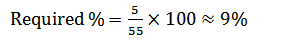Courses

# NABARD Quantitative Aptitude Practice: 2

## 30 Questions MCQ Test NABARD Assistant Manager Grade A Mock Test Series | NABARD Quantitative Aptitude Practice: 2

Description
This mock test of NABARD Quantitative Aptitude Practice: 2 for Banking Exams helps you for every Banking Exams entrance exam. This contains 30 Multiple Choice Questions for Banking Exams NABARD Quantitative Aptitude Practice: 2 (mcq) to study with solutions a complete question bank. The solved questions answers in this NABARD Quantitative Aptitude Practice: 2 quiz give you a good mix of easy questions and tough questions. Banking Exams students definitely take this NABARD Quantitative Aptitude Practice: 2 exercise for a better result in the exam. You can find other NABARD Quantitative Aptitude Practice: 2 extra questions, long questions & short questions for Banking Exams on EduRev as well by searching above.
QUESTION: 1

### What should in place of questions mark (?) in the questions given below?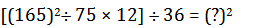Solution: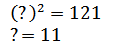QUESTION: 2

### What should in place of questions mark (?) in the questions given below?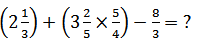Solution: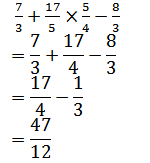QUESTION: 3

### What should in place of questions mark (?) in the questions given below?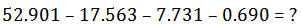Solution: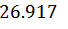QUESTION: 4

What should in place of questions mark (?) in the questions given below?

? % of 762 + 44% of 568 = 524.24

Solution: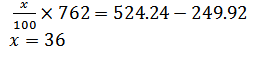QUESTION: 5

What should in place of questions mark (?) in the questions given below?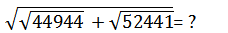Solution: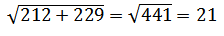QUESTION: 6

What should in place of question mark (?) in the following questions?

40280625, 732375, 16275, 465, 18.6, 1.24, ?

Solution: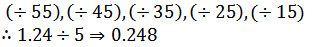QUESTION: 7

What should in place of question mark (?) in the following questions?

14, 12, 21, 59, 231, 1149, ?

Solution: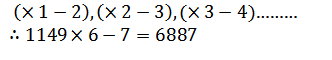QUESTION: 8

What should in place of question mark (?) in the following questions?

1728, 2744, 4096, 5832, 8000, 10648, ?

Solution: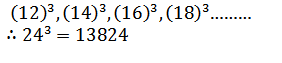QUESTION: 9

What should in place of question mark (?) in the following questions?
8, 10, 14.5, 22.5, 35, 53, ?

Solution: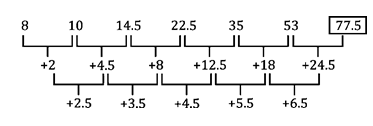QUESTION: 10

What should in place of question mark (?) in the following questions?

2, 28, 252, 1008, 4032, 36288, ?

Solution: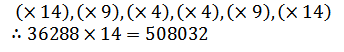QUESTION: 11

Kurti took a loan at simple interest rate of 6% in the first year with an increase of 0.5% in each subsequent year. She paid interest of Rs. 3375 after four years. How much loan did she takes?

Solution: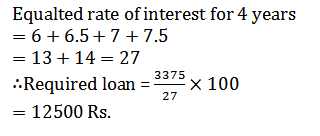QUESTION: 12

What would be the cost of building a fence around a circular plot of area 98.56 sq. ft, if the cost of fencing per foot is Rs. 614?

Solution: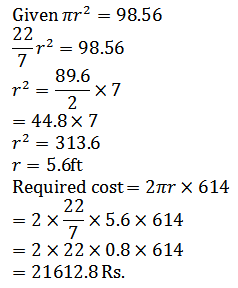QUESTION: 13

Aman’s expense is 30% more than Vimal’s expense and vimal’s expense is 10% less than Raman’s expense. If the sum of their expenses is Rs. 6447, then what would be the Aman’s expense?

Solution: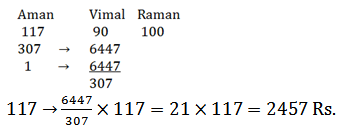QUESTION: 14

In a test, a candidate secured 336 marks out of maximum marks ‘x’. If the maximum marks ‘x’ were converted into 400 marks, he would have secured 192 marks. What were the maximum marks of the test?

Solution: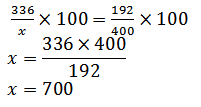QUESTION: 15

An AC consumes 8 units of electricity in 30 min and a bulb consumes 18 units of electricity in 6 h. How much total unit of electricity will both AC and bulb consume in 8 days if they run 10 h a day?

Solution: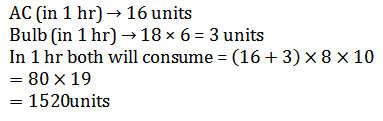QUESTION: 16

What amount a man would have received on a principal of Rs. 4000 after 2 yr compound interest at the rate of 5% per annum?

Solution: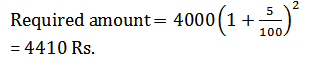QUESTION: 17

Four years ago shayam’s age was 3/4 times that of Ram. Four years hence, shayam’s age will be 5/6 times that of Ram. What is the present age of shayam?

Solution: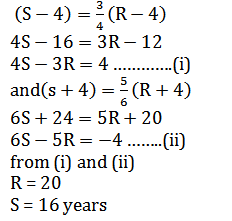QUESTION: 18

A bus started its Journey from Ramgarh and reached Devgarh in 44 min with its average speed of 50 km/h. If the average speed of the bus is increased by 5 km/h, how much time will it take to cover the same distance?

Solution: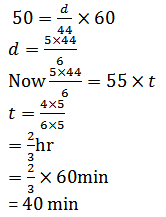QUESTION: 19

The average age of the family of five numbers of 24. If the present age of youngest member is 8 yr, then what was the average age of the family at the time of the birth of the youngest member?

Solution: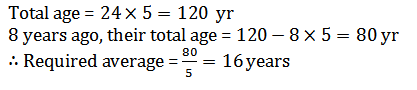QUESTION: 20

A candidate appearing for an examination has to secure 35% marks to pass. But he secured only 40 marks and failed by 30 marks. What would be the maximum marks of test?

Solution: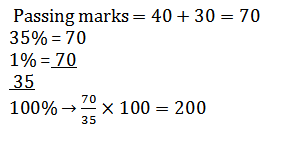QUESTION: 21

Study the following table and answer the questions given below.
Plan of public sectors under Various plan Sector-wise Expenditure out of the total Expenditure (in Rs. Millions)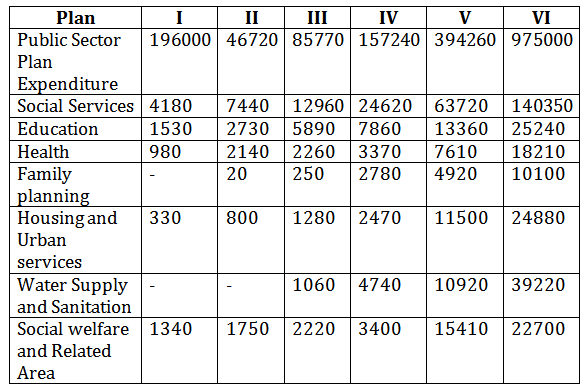Q. In various plans in the ratio of expenditure on Public Sector, which of the following graphs explain best the expenditure on Water Supply and Sanitation?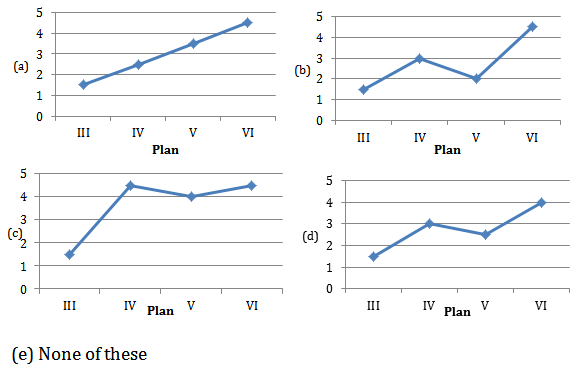Solution:

The expenditure increases consistently.

QUESTION: 22

Study the following table and answer the questions given below.
Plan of public sectors under Various plan Sector-wise Expenditure out of the total Expenditure (in Rs. Millions)Q. In various plans in the ratio of expenditure on Public Sector, which of the following graphs explain best the expenditure on Water Supply and Sanitation?Q. In the ratio of public Sector Expenditure the expenditure on Social Services was highest in which plan

Solution:

It is obvious from the table.

QUESTION: 23

Study the following table and answer the questions given below.
Plan of public sectors under Various plan Sector-wise Expenditure out of the total Expenditure (in Rs. Millions)Q. In various plans in the ratio of expenditure on Public Sector, which of the following graphs explain best the expenditure on Water Supply and Sanitation?Q. In the successive plan in the ratio of Public Sector Expenditure, there was a continuous decrease in which sector?

Solution:

Social Welfare & Related areas

QUESTION: 24

Study the following table and answer the questions given below.
Plan of public sectors under Various plan Sector-wise Expenditure out of the total Expenditure (in Rs. Millions)Q. In various plans in the ratio of expenditure on Public Sector, which of the following graphs explain best the expenditure on Water Supply and Sanitation?Q. For plan VI out of Public Sector Expenditure, what percent of expenditure is on Housing and Urban services?

Solution: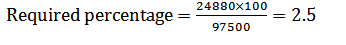QUESTION: 25

Study the following table and answer the questions given below.
Plan of public sectors under Various plan Sector-wise Expenditure out of the total Expenditure (in Rs. Millions)Q. In various plans in the ratio of expenditure on Public Sector, which of the following graphs explain best the expenditure on Water Supply and Sanitation?Q. For all the given plans, what was the difference in expenditure on Education and Health?

Solution:

Expenditure on Education = Rs (1530+2730+5890+7860+13360+25240) millions
= Rs. 56610 millions
Expenditure on health = Rs. (980+2140+2260+3370+7610+18210) Millions
= Rs. 34570 millions
∴ Difference = Rs. (56610 – 34570) millions
= Rs. 22040 million

QUESTION: 26

Study the graph carefully to answer the following questions.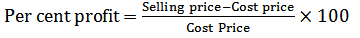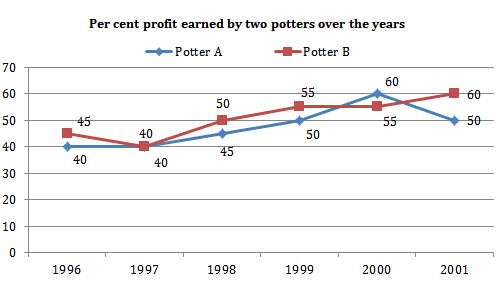Q. If potter A sold 3650 pots in the year 1999 at the price of Rs. 12 per pot, what is the cost price of all pots together?

Solution: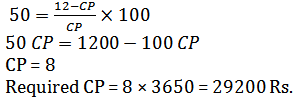QUESTION: 27

Study the graph carefully to answer the following questions.Q. If the amount of profit earned by potter B in the year 1996 is Rs. 40500, what was the selling price of all the pots in that year?

Solution: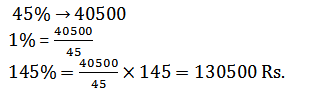QUESTION: 28

Study the graph carefully to answer the following questions.Q. If in the year 2000, the selling price of potter B is equal to the total cost price of potter A, what is the ratio of their selling prices?

Solution: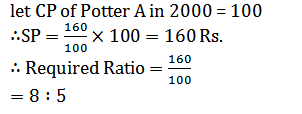QUESTION: 29

Study the graph carefully to answer the following questions.Q. Which of the following statement is/are definitely true?

Solution:

Amount is not given in any case.

QUESTION: 30

Study the graph carefully to answer the following questions.Q. What is the approximate per cent rise in per cent profit of potter B in the year 2001 from the previous year?

Solution: